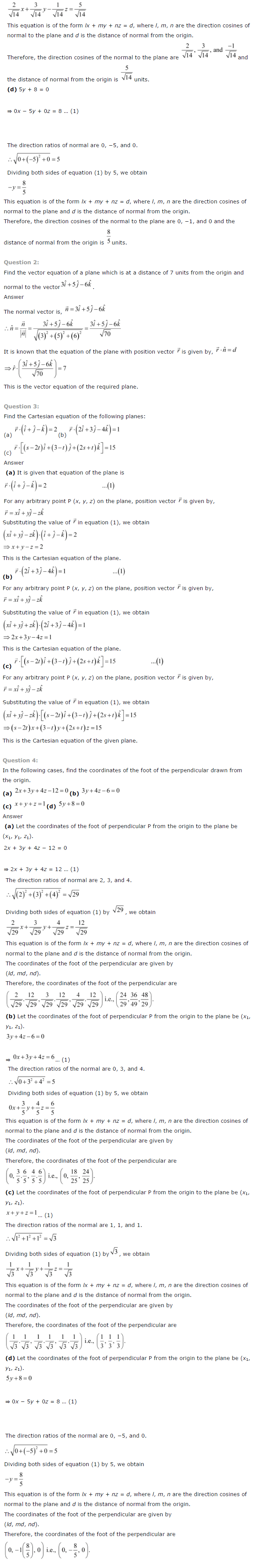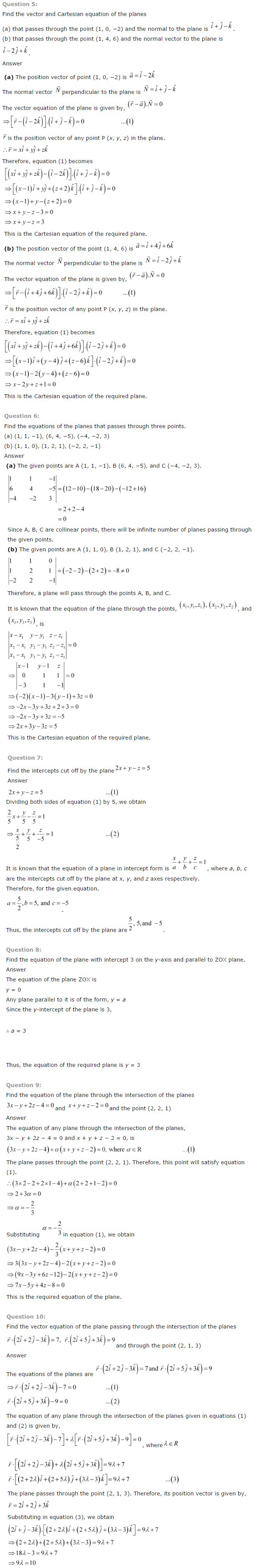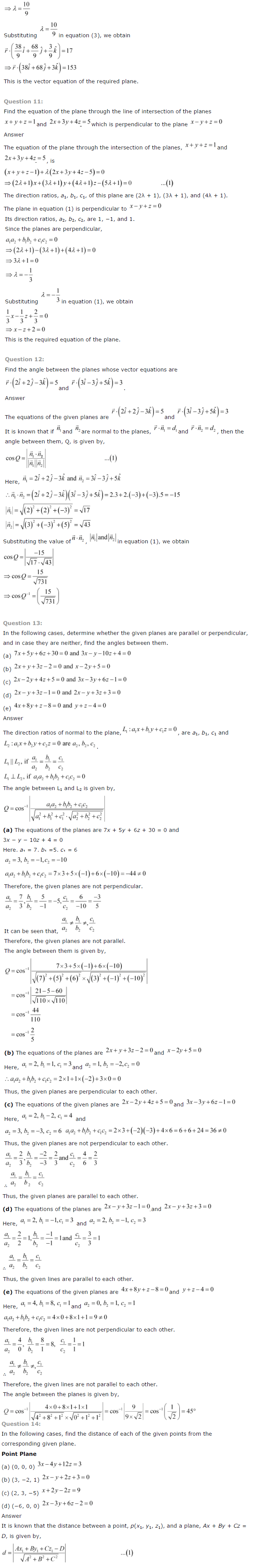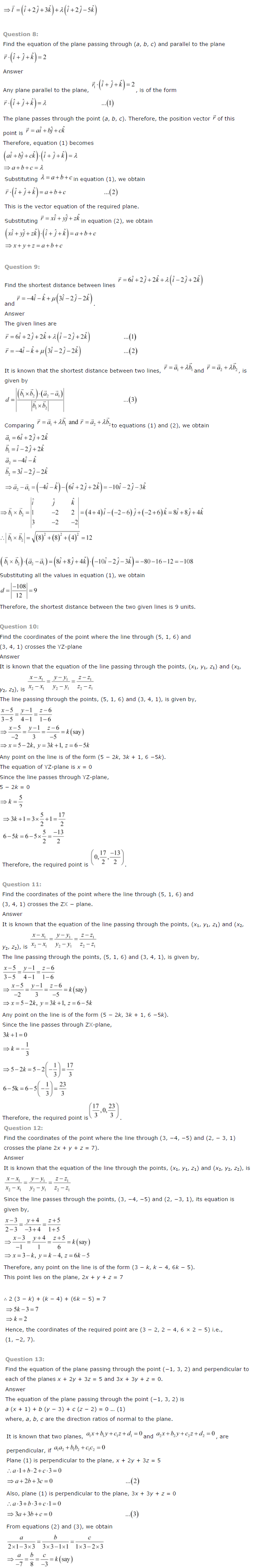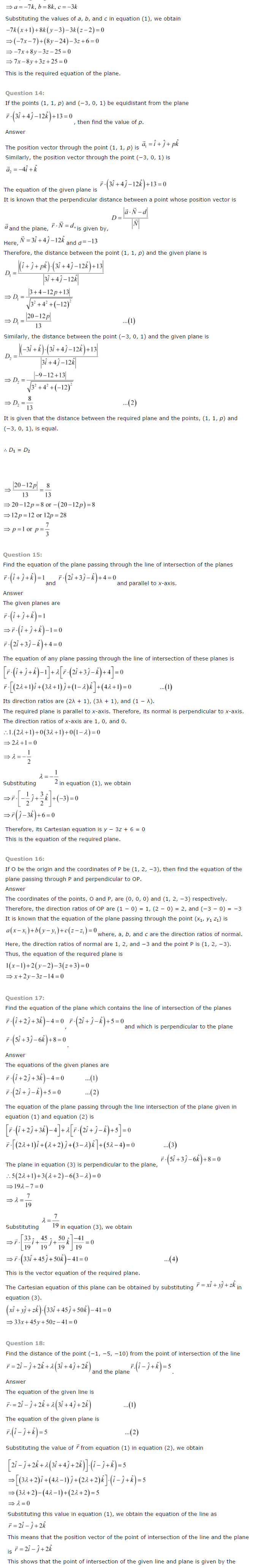# NCERT Solutions for Class 12 Maths Chapter 11 Three Dimensional Geometry

ncert textbook

## NCERT Solutions for Class 12 Maths Chapter 11 Three Dimensional Geometry

NCERT Solutions for Class 12 Maths – Chapter 11 – Three Dimensional Geometry– is designed and prepared by the best teachers across India. All the important topics are covered in the exercises and each answer comes with a detailed explanation to help students understand concepts better. These NCERT solutions play a crucial role in your preparation for all exams conducted by the CBSE, including the JEE.

Chapter 11 – Three Dimensional Geometry covers multiple exercises. The answer to each question in every exercise is provided along with complete, step-wise solutions for your better understanding. This will prove to be most helpful to you in your home assignments as well as practice sessions.
The topics and sub-topics included in the Three Dimensional Geometry chapter are the following:

 Section Name Topic Name 11 Three Dimensional Geometry 11.1 Introduction 11.2 Direction Cosines and Direction Ratios of a Line 11.3 Equation of a Line in Space 11.4 Angle between Two Lines 11.5 Shortest Distance between Two Lines 11.6 Plane 11.7 Coplanarity of Two Lines 11.8 Angle between Two Lines 11.9 Distance of a Point from a P 11.10 Angle between a L ine and a Plane